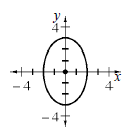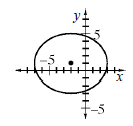### Home > A2C > Chapter 11 > Lesson 11.2.5 > Problem11-99

11-99.

Graph each of the following ellipses.

1. $\frac { x ^ { 2 } } { 4 } + \frac { y ^ { 2 } } { 9 } = 1$1. $\frac { ( x + 2 ) ^ { 2 } } { 25 } + \frac { ( y - 1 ) ^ { 2 } } { 16 } = 1$